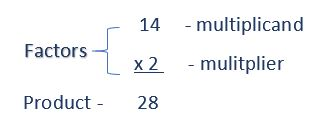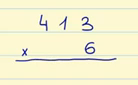Smartick is a fun way to learn math!Apr13

# What is Multiplication: Steps to Learn and Understand Multiplication

What is multiplication? We will explain it in this post! Then we will show some examples using the steps that you should follow to complete multiplication problems, along with a video.

Index

## What is Multiplication?

Multiplication is a mathematical operation that is performed to calculate the result by adding the number as many times as indicated by the other number in the operation, for example:

52 × 4 = 208

We can express this operation as:

52 + 52 + 52 + 52 = 208

As you can see we added the number 52 four times, the equivalent of multiplying it by 4. A bit time-consuming. And this is only when multiplying by 4, imagine multiplying it by 235, or 8128. If we didn’t know how to multiply then we would have to add it many times. Multiplying is a more effective operation than adding the same number many times.

The word multiplication comes from the Latin word multiplicare which means ”to increase”. Look closely at the word “multiplication”, it has the root “multi-” the same that is used in “multiple” or “multitude”, which means a lot of something.

For example, six friends want to know how many candies they have in total if they put them together. Each one has  5 candies in their bag. How many candies do they have in total?

• The six friends can add: 5 + 5 + 5 + 5 + 5 + 5 = 30, but this is a little repetitive.
• So they decide to multiply 5 candies by the 6 bags that they have in total.
• 5 candies multiplied by 6 bags is equal to the 30 candies that they have between all of them.

## Multiplication Terms

They are all of the numbers that are involved in multiplication, from the factors – multiplicand and multiplier – to the product.

### Factors

Factors are the numbers that are multiplied.

The factors are written one under another. Normally the larger number is written above, the one we are going to multiply, which is why we call it the multiplicand. Underneath is the smaller number, which indicates the number of times we repeat the multiplication, we call it the multiplier.### Product

Product is the result of the multiplication.

## Multiplication StepsKeeping in mind the example in the image above, these are the steps to solve the multiplication:

1. Multiply the multiplier (the number below, 6) by each of the digits of the multiplicand (the number above, 413), from right to left, beginning with 3 x 6.
2. The units of the products are written below the line, vertically in line with the units being multiplied (below the 6). Since 3 x 6 is 18, we have 18 units, so we write an 8 and save the 1 to add to the tens.
3. The tens of the product are below the tens being multiplied. We have saved one which we have “carried over” from the previous step. Since 1 x 6 is equal to 6, plus 1 which we carried over, we have 7. The digit for the tens will be 7, and we don’t carry anything to the next step.
4. Now we will multiply 6 by the 4 hundreds, giving us 24 hundreds. We put the 4 below the tens, and since we have nothing left to multiply, we put 2 to the left of the 4. 24 hundreds are 2 thousands and 4 hundreds.
5. The result of multiplying 413 by 6 is 2478. In other words 2 thousands, 4 hundreds, 7 tens, and 8 units. You can review all of these terms in our post about units, tens, and hundreds.

## Multiplication Example

Now that you know how to solve a multiplication problem, we are going to look at another example in a little more detail.

For example, we are going to multiply 561 × 3.

We start by multiplying 1 × 3 = 3.

We write the 3 below the line, in the same position as the units.

Now we multiply 6 × 3 = 18.

Since we are multiplying 6 tens by 3 we get 18 tens. The 8 tens we write under the line, in the tens position and a hundred, which we save to add during the next step.

Finally, we multiply 5 × 3  = 15.

15 hundreds, plus 1 from the previous step gives us 16. And since there is nothing else to multiply we write 16 below the line.

And the product of this multiplication is 561 × 3 = 1,683.

Look closely at the hundred that we saved and put above the 5 so that we did not forget it.

If you would like you can refresh your memory and learn about the multiplication tables with some tricks to memorize them more quickly.

## Video to Learn How to Multiply by 2 Digits

What is multiplication? What about 2 digit multiplication? Take a look at the following video to better understand multiplication by 2 digits. This is a video of one of our interactive tutorials and although it is no longer interactive you have the advantage of watching it as many times as you need and are able to share it with friends.

If you would like to access our interactive tutorials, register with Smartick! The online method which helps children ages 4 to 14 learn and practice math.